# 神经网络变得轻松（第三部分）：卷积网络

### 内容

• 概述
• 1. 卷积神经网络的显著特征
• 1.1. 卷积层
• 1.2. 子抽样层
• 2. 在卷积层中训练神经元的原理
• 3. 建立卷积神经网络
• 3.1. 神经元基类
• 3.1.1. 前馈
• 3.1.2. 误差梯度计算
• 3.2. 子抽样层元素
• 3.2.1. 前馈
• 3.2.2. 误差梯度计算
• 3.3. 卷积层元素
• 3.4. 创建卷积神经网络类
• 3.4.1. 卷积神经网络类构造函数
• 3.4.2. 卷积神经网络前向传播方法
• 3.4.3. 卷积神经网络后向传播方法
• 4. 测试
• 结束语
• 参考文献列表
• 本文中用到的程序

### 1. 卷积神经网络的显著特征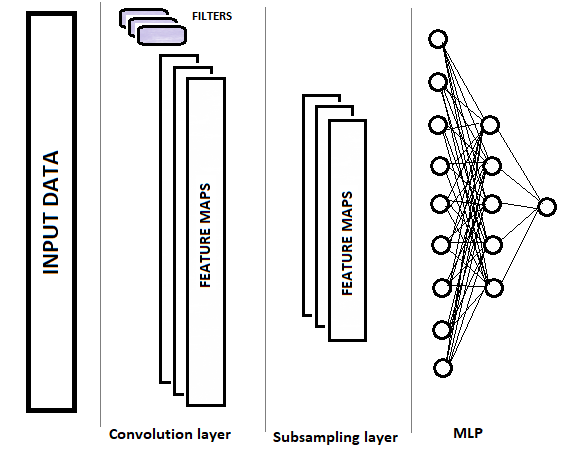#### 1.1. 卷积层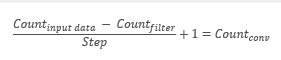### 3. 建立卷积神经网络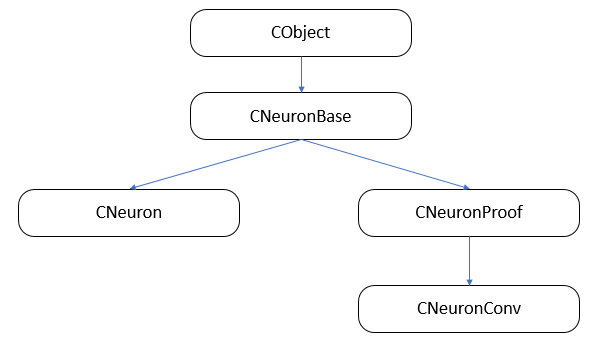#### 3.1. 神经元的基类。

```class CNeuronBase    :  public CObject
{
protected:
double            eta;
double            alpha;
double            outputVal;
uint              m_myIndex;
CArrayCon        *Connections;
//---
virtual bool      feedForward(CLayer *prevLayer)               {  return false;     }
virtual bool      calcHiddenGradients( CLayer *&nextLayer)     {  return false;     }
virtual bool      updateInputWeights(CLayer *&prevLayer)       {  return false;     }
virtual double    activationFunction(double x)                 {  return 1.0;       }
virtual double    activationFunctionDerivative(double x)       {  return 1.0;       }
virtual CLayer    *getOutputLayer(void)                        {  return NULL;      }
public:
CNeuronBase(void);
~CNeuronBase(void);
virtual bool      Init(uint numOutputs, uint myIndex);
//---
virtual void      setOutputVal(double val)                     {  outputVal=val;    }
virtual double    getOutputVal()                               {  return outputVal; }
//---
virtual bool      feedForward(CObject *&SourceObject);
virtual bool      updateInputWeights(CObject *&SourceObject);
//---
virtual bool      Save( int const file_handle);
//---
virtual int       Type(void)        const                       {  return defNeuronBase;                  }
};
```

#### 3.1.1. 前馈。

```bool CNeuronBase::feedForward(CObject *&SourceObject)
{
bool result=false;
//---
if(CheckPointer(SourceObject)==POINTER_INVALID)
return result;
```

```   CLayer *temp_l;
CNeuronProof *temp_n;

```

```   switch(SourceObject.Type())
{
case defLayer:
temp_l=SourceObject;
result=feedForward(temp_l);
break;
case defNeuronConv:
case defNeuronProof:
temp_n=SourceObject;
result=feedForward(temp_n.getOutputLayer());
break;
}
//---
return result;
}
```

#### 3.1.2. 误差梯度计算。

```bool CNeuronBase::calcHiddenGradients(CObject *&TargetObject)
{
bool result=false;
//---
if(CheckPointer(TargetObject)==POINTER_INVALID)
return result;
//---
CLayer *temp_l;
CNeuronProof *temp_n;
switch(TargetObject.Type())
{
case defLayer:
temp_l=TargetObject;
break;
case defNeuronConv:
case defNeuronProof:
switch(Type())
{
case defNeuron:
temp_n=TargetObject;
break;
default:
temp_n=GetPointer(this);
temp_l=temp_n.getOutputLayer();
temp_n=TargetObject;
break;
}
break;
}
//---
return result;
}
```

#### 3.2. 子抽样层元素。

```class CNeuronProof : public CNeuronBase
{
protected:
CLayer            *OutputLayer;
int               iWindow;
int               iStep;

virtual bool      feedForward(CLayer *prevLayer);

public:
CNeuronProof(void){};
~CNeuronProof(void);
virtual bool      Init(uint numOutputs,uint myIndex,int window, int step, int output_count);
//---
virtual CLayer   *getOutputLayer(void)  { return OutputLayer;  }
virtual bool      calcInputGradients( CLayer *prevLayer) ;
virtual bool      calcInputGradients( CNeuronBase *prevNeuron, uint index) ;
//--- methods for working with files
virtual bool      Save( int const file_handle)                         { return(CNeuronBase::Save(file_handle) && OutputLayer.Save(file_handle));   }
virtual int       Type(void)   const   {  return defNeuronProof;   }
};
```

#### 3.2.1. 前馈。

feedForward 方法用于滤除噪声，并减小特征数组的尺寸。 在所述的解决方案中，算术平均函数用于压缩数据。 我们来更详尽地研究该方法的代码。 在该方法伊始，检查指向神经元前一层指针的相关性。

```bool CNeuronProof::feedForward(CLayer *prevLayer)
{
if(CheckPointer(prevLayer)==POINTER_INVALID)
return false;
```

```   int total=prevLayer.Total()-iWindow+1;
CNeuron *temp;
for(int i=0;(i<=total && result);i+=iStep)
{
```

```      double sum=0;
for(int j=0;j<iWindow;j++)
{
temp=prevLayer.At(i+j);
if(CheckPointer(temp)==POINTER_INVALID)
continue;
sum+=temp.getOutputVal();
}
```

```      temp=OutputLayer.At(i/iStep);
if(CheckPointer(temp)==POINTER_INVALID)
return false;
temp.setOutputVal(sum/iWindow);
}
//---
return true;
}
```

#### 3.2.2. 误差梯度计算。

```bool CNeuronProof::calcHiddenGradients( CLayer *&nextLayer)
{
if(CheckPointer(nextLayer)==POINTER_INVALID || CheckPointer(OutputLayer)==POINTER_INVALID || OutputLayer.Total()<=0)
return false;
```

```   gradient=0;
int total=OutputLayer.Total();
CNeuron *temp;
for(int i=0;i<total;i++)
{
temp=OutputLayer.At(i);
if(CheckPointer(temp)==POINTER_INVALID)
return false;
}
//---
return true;
}
```

```bool CNeuronProof::calcInputGradients(CLayer *prevLayer)
{
if(CheckPointer(prevLayer)==POINTER_INVALID || CheckPointer(OutputLayer)==POINTER_INVALID)
return false;
```

```   if(prevLayer.At(0).Type()!=defNeuron)
{
CNeuronProof *temp=prevLayer.At(m_myIndex);
if(CheckPointer(temp)==POINTER_INVALID)
return false;
prevLayer=temp.getOutputLayer();
if(CheckPointer(prevLayer)==POINTER_INVALID)
return false;
}
```

```   CNeuronBase *prevNeuron, *outputNeuron;
int total=prevLayer.Total();
for(int i=0;i<total;i++)
{
prevNeuron=prevLayer.At(i);
if(CheckPointer(prevNeuron)==POINTER_INVALID)
continue;
```

```      double prev_gradient=0;
int start=i-iWindow+iStep;
start=(start-start%iStep)/iStep;
double stop=(i-i%iStep)/iStep+1;
```

```      for(int out=(int)fmax(0,start);out<(int)fmin(OutputLayer.Total(),stop);out++)
{
outputNeuron=OutputLayer.At(out);
if(CheckPointer(outputNeuron)==POINTER_INVALID)
continue;
}
}
//---
return true;
}
```

#### 3.3. 卷积层元素。

```class CNeuronConv  :  public CNeuronProof
{
protected:
double            param;   //PReLU param
virtual bool      feedForward(CLayer *prevLayer);
virtual double    activationFunction(double x);
virtual bool      updateInputWeights(CLayer *&prevLayer);
public:
CNeuronConv() :   param(0.01) { };
~CNeuronConv(void)             { };
//---
virtual bool      calcInputGradients(CNeuronBase *prevNeuron, uint index) ;
virtual double    activationFunctionDerivative(double x);
virtual int       Type(void)   const   {  return defNeuronConv;   }
};
```

```bool CNeuronConv::updateInputWeights(CLayer *&prevLayer)
{
if(CheckPointer(prevLayer)==POINTER_INVALID || CheckPointer(OutputLayer)==POINTER_INVALID)
return false;
```

```   CConnection *con;
for(int n=0; n<iWindow && !IsStopped(); n++)
{
con=Connections.At(n);
if(CheckPointer(con)==POINTER_INVALID)
continue;
```

• 输入数据数组的第一个元素（偏移的步骤编号等于权重的序数）乘以误差梯度数组的最后一个元素，
• 输入数据数组的第二个元素（偏移的步骤编号等于权重的序数）乘以误差梯度数组的倒数第二个元素，
• 依此类推，直到索引指定的元素等于与内层数组中元素编号相等，且步骤编号等于权重序数，乘以误差梯度数组的第一个元素。

```      double delta=0;
int total_i=OutputLayer.Total();
CNeuron *prev, *out;
for(int i=0;i<total_i;i++)
{
prev=prevLayer.At(n*iStep+i);
out=OutputLayer.At(total_i-i-1);
if(CheckPointer(prev)==POINTER_INVALID || CheckPointer(out)==POINTER_INVALID)
continue;
}
```

```      con.weight+=con.deltaWeight=(delta!=0 ? eta*delta : 0)+(con.deltaWeight!=0 ? alpha*con.deltaWeight : 0);
}
//---
return true;
}
```

#### 3.4. 创建一个卷积神经网络类。

```class CLayerDescription    :  public CObject
{
public:
CLayerDescription(void);
~CLayerDescription(void){};
//---
int               type;
int               count;
int               window;
int               step;
};
//+------------------------------------------------------------------+
//|                                                                  |
//+------------------------------------------------------------------+
CLayerDescription::CLayerDescription(void)   :  type(defNeuron),
count(0),
window(1),
step(1)
{}
```

• layers — 一个层数组;
• recentAverageError  — 当前网络误差;
• recentAverageSmoothingFactor  — 误差平均因子;
• CNetConvolution  — 类构造器;
• ~CNetConvolution  — 类析构函数;
• feedForward  — 前行推算方法;
• backProp  — 回测推算方法;
• getResults  — 获取最后一次前行推算结果的方法;
• getRecentAverageError  — 获取当前网络误差的方法;
• Save and Load — 保存和加载先前创建和训练结果的方法。

```class CNetConvolution
{
public:
CNetConvolution(CArrayObj *Description);
~CNetConvolution(void)                     {  delete layers; }
bool              feedForward( CArrayDouble *inputVals);
void              backProp( CArrayDouble *targetVals);
void              getResults(CArrayDouble *&resultVals) ;
double            getRecentAverageError()                   { return recentAverageError; }
bool              Save( string file_name, double error, double undefine, double forecast, datetime time, bool common=true);
bool              Load( string file_name, double &error, double &undefine, double &forecast, datetime &time, bool common=true);
//---
static double     recentAverageSmoothingFactor;
virtual int       Type(void)   const   {  return defNetConv;   }

private:
CArrayLayer       *layers;
double            recentAverageError;
};
```

#### 3.4.1. 卷积神经网络类构造函数。

```CNetConvolution::CNetConvolution(CArrayObj *Description)
{
if(CheckPointer(Description)==POINTER_INVALID)
return;
//---
int total=Description.Total();
if(total<=0)
return;
//---
layers=new CArrayLayer();
if(CheckPointer(layers)==POINTER_INVALID)
return;
```

```   CLayer *temp;
CLayerDescription *desc=NULL, *next=NULL, *prev=NULL;
CNeuronBase *neuron=NULL;
CNeuronProof *neuron_p=NULL;
int output_count=0;
int temp_count=0;
```

```   for(int i=0;i<total;i++)
{
prev=desc;
desc=Description.At(i);
if((i+1)<total)
{
next=Description.At(i+1);
if(CheckPointer(next)==POINTER_INVALID)
return;
}
else
next=NULL;
```

```      int outputs=(next==NULL || next.type!=defNeuron ? 0 : next.count);
temp=new CLayer(outputs);
```

```      for(int n=0;n<(desc.count+(i>0 && desc.type==defNeuron ? 1 : 0));n++)
{
switch(desc.type)
{
case defNeuron:
neuron=new CNeuron();
if(CheckPointer(neuron)==POINTER_INVALID)
{
delete temp;
delete layers;
return;
}
neuron.Init(outputs,n);
break;
```

```            case defNeuronConv:
neuron_p=new CNeuronConv();
if(CheckPointer(neuron_p)==POINTER_INVALID)
{
delete temp;
delete layers;
return;
}
if(CheckPointer(prev)!=POINTER_INVALID)
{
if(prev.type==defNeuron)
{
temp_count=(int)((prev.count-desc.window)%desc.step);
output_count=(int)((prev.count-desc.window-temp_count)/desc.step+(temp_count==0 ? 1 : 2));
}
else
if(n==0)
{
temp_count=(int)((output_count-desc.window)%desc.step);
output_count=(int)((output_count-desc.window-temp_count)/desc.step+(temp_count==0 ? 1 : 2));
}
}
if(neuron_p.Init(outputs,n,desc.window,desc.step,output_count))
neuron=neuron_p;
break;
```

```            case defNeuronProof:
neuron_p=new CNeuronProof();
if(CheckPointer(neuron_p)==POINTER_INVALID)
{
delete temp;
delete layers;
return;
}
if(CheckPointer(prev)!=POINTER_INVALID)
{
if(prev.type==defNeuron)
{
temp_count=(int)((prev.count-desc.window)%desc.step);
output_count=(int)((prev.count-desc.window-temp_count)/desc.step+(temp_count==0 ? 1 : 2));
}
else
if(n==0)
{
temp_count=(int)((output_count-desc.window)%desc.step);
output_count=(int)((output_count-desc.window-temp_count)/desc.step+(temp_count==0 ? 1 : 2));
}
}
if(neuron_p.Init(outputs,n,desc.window,desc.step,output_count))
neuron=neuron_p;
break;
}
```

```         if(!temp.Add(neuron))
{
delete temp;
delete layers;
return;
}
neuron=NULL;
}
```

```      if(!layers.Add(temp))
{
delete temp;
delete layers;
return;
}
}
//---
return;
}
```

#### 3.4.2. 卷积神经网络前行传播方法。

```bool CNetConvolution::feedForward(CArrayDouble *inputVals)
{
if(CheckPointer(layers)==POINTER_INVALID || CheckPointer(inputVals)==POINTER_INVALID || layers.Total()<=1)
return false;
```

```   CLayer *previous=NULL;
CLayer *current=layers.At(0);
int total=MathMin(current.Total(),inputVals.Total());
CNeuronBase *neuron=NULL;
for(int i=0;i<total;i++)
{
neuron=current.At(i);
if(CheckPointer(neuron)==POINTER_INVALID)
return false;
neuron.setOutputVal(inputVals.At(i));
}
```

```   CObject *temp=NULL;
for(int l=1;l<layers.Total();l++)
{
previous=current;
current=layers.At(l);
if(CheckPointer(current)==POINTER_INVALID)
return false;
```

```      total=current.Total();
if(current.At(0).Type()==defNeuron)
total--;
//---
for(int n=0;n<total;n++)
{
neuron=current.At(n);
if(CheckPointer(neuron)==POINTER_INVALID)
return false;
```

```         if(previous.At(0).Type()==defNeuron)
{
temp=previous;
if(!neuron.feedForward(temp))
return false;
continue;
}
```

```         if(neuron.Type()==defNeuron)
{
if(n==0)
{
CLayer *temp_l=new CLayer(total);
if(CheckPointer(temp_l)==POINTER_INVALID)
return false;
CNeuronProof *proof=NULL;
for(int p=0;p<previous.Total();p++)
{
proof=previous.At(p);
if(CheckPointer(proof)==POINTER_INVALID)
return false;
}
temp=temp_l;
}
if(!neuron.feedForward(temp))
return false;
if(n==total-1)
{
CLayer *temp_l=temp;
temp_l.FreeMode(false);
temp_l.Shutdown();
delete temp_l;
}
continue;
}
```

```         temp=previous.At(n);
if(CheckPointer(temp)==POINTER_INVALID)
return false;
if(!neuron.feedForward(temp))
return false;
}
}
//---
return true;
}
```

#### 3.4.3. 卷积神经网络回退传播方法。

```void CNetConvolution::backProp(CArrayDouble *targetVals)
{
if(CheckPointer(targetVals)==POINTER_INVALID)
return;
```

```   CLayer *outputLayer=layers.At(layers.Total()-1);
if(CheckPointer(outputLayer)==POINTER_INVALID)
return;
//---
double error=0.0;
int total=outputLayer.Total()-1;
for(int n=0; n<total && !IsStopped(); n++)
{
CNeuron *neuron=outputLayer.At(n);
double target=targetVals.At(n);
double delta=(target>1 ? 1 : target<-1 ? -1 : target)-neuron.getOutputVal();
error+=delta*delta;
}
error/= total;
error = sqrt(error);

recentAverageError+=(error-recentAverageError)/recentAverageSmoothingFactor;
```

下一步是组织所有神经网络层的回退循环。 在此，我们运行一个嵌套循环遍历相应层的所有神经元，以便重新计算隐藏层神经元的误差梯度。

```   CNeuronBase *neuron=NULL;
CObject *temp=NULL;
for(int layerNum=layers.Total()-2; layerNum>0; layerNum--)
{
CLayer *hiddenLayer=layers.At(layerNum);
CLayer *nextLayer=layers.At(layerNum+1);
total=hiddenLayer.Total();
for(int n=0; n<total && !IsStopped(); ++n)
{
```

```         neuron=hiddenLayer.At(n);
if(nextLayer.At(0).Type()==defNeuron)
{
temp=nextLayer;
continue;
}
```

```         if(neuron.Type()==defNeuron)
{
double g=0;
for(int i=0;i<nextLayer.Total();i++)
{
temp=nextLayer.At(i);
}
continue;
}
temp=nextLayer.At(n);
}
}
```

```   for(int layerNum=layers.Total()-1; layerNum>0; layerNum--)
{
CLayer *layer=layers.At(layerNum);
CLayer *prevLayer=layers.At(layerNum-1);
total=layer.Total()-(layer.At(0).Type()==defNeuron ? 1 : 0);
int n_conv=0;
for(int n=0; n<total && !IsStopped(); n++)
{
neuron=layer.At(n);
if(CheckPointer(neuron)==POINTER_INVALID)
return;
if(neuron.Type()==defNeuronProof)
continue;
switch(prevLayer.At(0).Type())
{
case defNeuron:
temp=prevLayer;
neuron.updateInputWeights(temp);
break;
case defNeuronConv:
case defNeuronProof:
if(neuron.Type()==defNeuron)
{
for(n_conv=0;n_conv<prevLayer.Total();n_conv++)
{
temp=prevLayer.At(n_conv);
neuron.updateInputWeights(temp);
}
}
else
{
temp=prevLayer.At(n);
neuron.updateInputWeights(temp);
}
break;
default:
temp=NULL;
break;
}
}
}
}
```

### 4. 测试

EA 本身仅需很少的修改。 该修改适用于神经网络类，即变量的声明和实例的创建。

`CNetConvolution     *Net;`

```   Net=new CNetConvolution(NULL);
ResetLastError();
{
printf("%s - %d -> Error of read %s prev Net %d",__FUNCTION__,__LINE__,FileName+".nnw",GetLastError());
CArrayObj *Topology=new CArrayObj();
if(CheckPointer(Topology)==POINTER_INVALID)
return INIT_FAILED;
//---
CLayerDescription *desc=new CLayerDescription();
if(CheckPointer(desc)==POINTER_INVALID)
return INIT_FAILED;
desc.count=(int)HistoryBars*12;
desc.type=defNeuron;
return INIT_FAILED;
//---
int filters=4;
desc=new CLayerDescription();
if(CheckPointer(desc)==POINTER_INVALID)
return INIT_FAILED;
desc.count=filters;
desc.type=defNeuronConv;
desc.window=12;
desc.step=12;
return INIT_FAILED;
//---
desc=new CLayerDescription();
if(CheckPointer(desc)==POINTER_INVALID)
return INIT_FAILED;
desc.count=filters;
desc.type=defNeuronProof;
desc.window=3;
desc.step=2;
return INIT_FAILED;
//---
int n=1000;
bool result=true;
for(int i=0;(i<4 && result);i++)
{
desc=new CLayerDescription();
if(CheckPointer(desc)==POINTER_INVALID)
return INIT_FAILED;
desc.count=n;
desc.type=defNeuron;
n=(int)MathMax(n*0.3,20);
}
if(!result)
{
delete Topology;
return INIT_FAILED;
}
//---
desc=new CLayerDescription();
if(CheckPointer(desc)==POINTER_INVALID)
return INIT_FAILED;
desc.count=3;
desc.type=defNeuron;
return INIT_FAILED;
delete Net;
Net=new CNetConvolution(Topology);
delete Topology;
if(CheckPointer(Net)==POINTER_INVALID)
return INIT_FAILED;
dError=-1;
dUndefine=0;
dForecast=0;
dtStudied=0;
}
```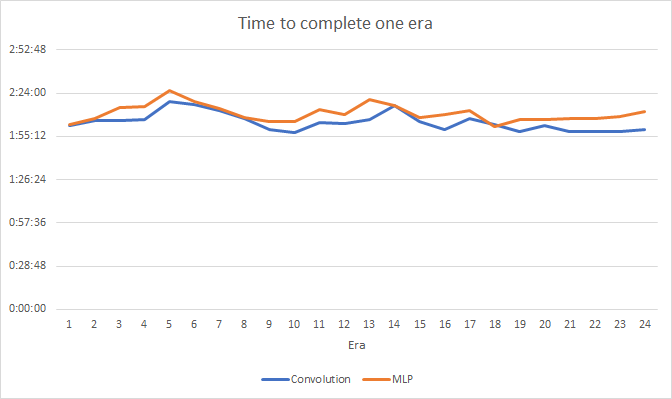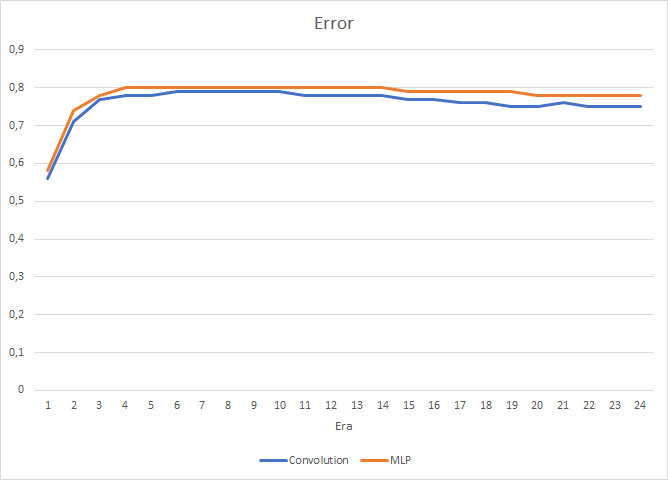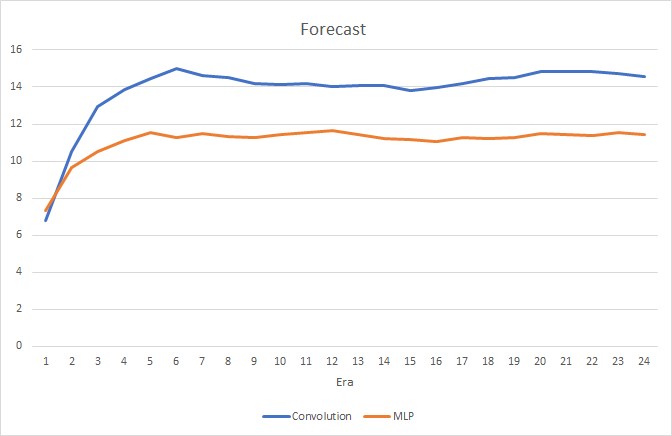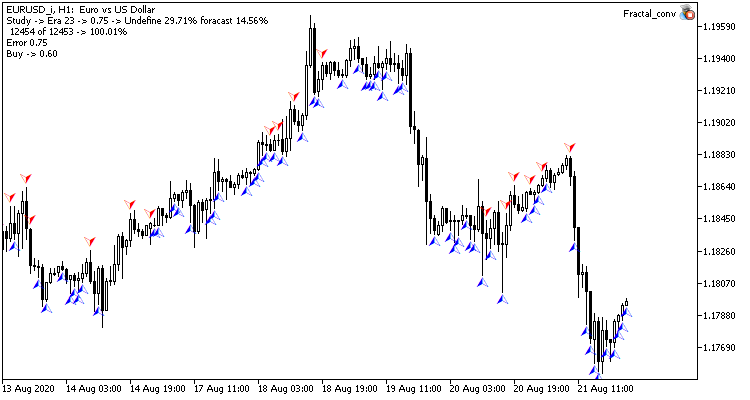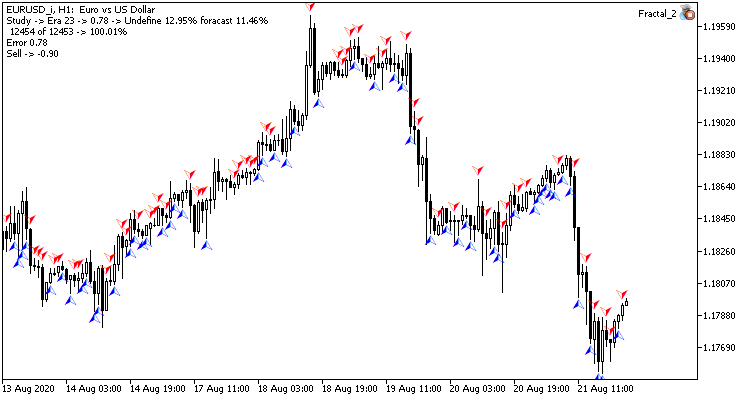### 参考文献列表

1. 神经网络变得轻松
2. 神经网络变得轻松（第二部分）：网络训练和测试

### 本文中用到的程序

# 发行 类型 说明
1 Fractal.mq5   智能交易系统  一款含有回归神经网络（输出层中有 1 个神经元）的智能交易系统
2 Fractal_2.mq5  智能交易系统  一款含有分类神经网络的智能交易系统（输出层中有 3 个神经元）
3 NeuroNet.mqh  类库  创建神经网络（感知器）的类库
4 Fractal_conv.mq5   智能交易系统  含有卷积神经网络（输出层中 3 个神经元）的神经智能交易系统

## 量化课程

移动端课程